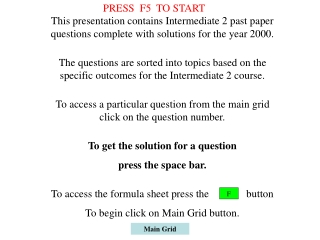DownloadDownload PresentationMain Grid

# Main Grid

Download Presentation## Main Grid

- - - - - - - - - - - - - - - - - - - - - - - - - - - E N D - - - - - - - - - - - - - - - - - - - - - - - - - - -
##### Presentation Transcript

1. PRESS F5 TO START This presentation contains Intermediate 2 past paper questions complete with solutions for the year 2000. The questions are sorted into topics based on the specific outcomes for the Intermediate 2 course. To access a particular question from the main grid click on the question number. To get the solution for a question press the space bar. To access the formula sheet press the button To begin click on Main Grid button. F Main Grid

2. Formulae List START Page

3. This is the formula that we use

4. 2000 Paper 1 Main Grid Solution

5. Intercept = 10 c = 10 10 5 Equation y= -2x + 10 Main Grid Solution

6. Main Grid Solution

7. Triangle in semi circle is right angled 25° Angles in triangle sum to 180° 65° Tangent to circle = 90° 25° BAC = 25° Solution Main Grid

8. Main Grid Solution

9. (a) 58 59 59 60 60 61 61 62 62 64 65 Q2 Q3 Q1 Position of median Q2= (11 + 1)/2 = 6th number Lower Quartile is 59 Upper Quartile is 62 (b) The median is 61 which is slightly above the average contents of 60. IQR is 3 (c) The median of 58 is well below the average contents of 60. IQR is 4 so much more varied amounts could be expected in the boxes too Main Grid

10. (x,y) for (5, 50) y = ax² 50 = a x 5² a = 50 ÷ 25 a = 2 Main Grid Solution

11. Period = 360 ÷ 2 = 180 So b = 2 Main Grid Solution

12. Solution Main Grid

13. 2000 P2 Main Grid Solution

14. Main Grid Solution

15. 19.4 Width = radius of table + 19.4 = 40 +19.4 = 59.4 cm Pythagoras x² = 40² - 35² x =√375 = 19.4 cm 35 x 40 Main Grid Solution

16. Amount due end of yr =£1000 x 1.185 =£1185 Cost of loan = £185 For 3 mths = £185 ÷4 =£46.25 £1000 x 1.015³ = £1045.67 Cost of 3 mth loan =£45.67 Advantage is cheaper by £0.58 Main Grid Solution

17. Main Grid Solution

18. (a) 3x + 50y = 88.50 Eq 1 x4 (b) 4x + 60y = 113.00 Eq 2 x3 12x + 200y = 354 12x + 180y = 339 Subtract200y – 180y = 354 – 339 20y = 15 y = 15 ÷20 = 0.75 Put into Eq 1 3x + 50 x 0.75 = 88.50 3x = 88.50 – 37.5 3x = 51 x = 17 Check with Eq 2 4 x 17 + 50 x 0.75 = 113 Car hire is £17 a day Fuel costs £0.75 per litre Main Grid

19. Main Grid Solution

20. Area of cross section = (20 x 10) + ¼ x ∏ x 20² = 200 + 314 = 514 cm² Volume = Ah = 514 x 42 = 21 588 =21 600 cm³ (3 sig figs) Volumes are the same, so the area of cross sections are equal since height is the same. Both have same rectangle 20 x 10. So area of area of triangle = ¼circle = 314 cm² So ½ x base x 20 = 314 Base x 20 = 628 Base = 628 ÷ 20 = 31.4 x = 31.4cm Main Grid

21. Main Grid Solution

22. D Adj 35m SOH CAH TOA 10° 35.5m 10° A Hyp 34m d B Use Cosine rule Main Grid

23. Main Grid Solution

24. Arc AC = arc BC = Perimeter AB = One circumference =∏ x D = 3.14 x 8 = 25.12 cm Total perimeter = 25.12 + 2 x 16.75 = 58.62 cm Main Grid

25. Main Grid Solution

26. B9 Main Grid Solution

27. 3 3 x = 3 x = 3 B(6, 11) (3, 20) Main Grid Solution

28. √ S A T C Main Grid Solution

29. Main Grid Solution